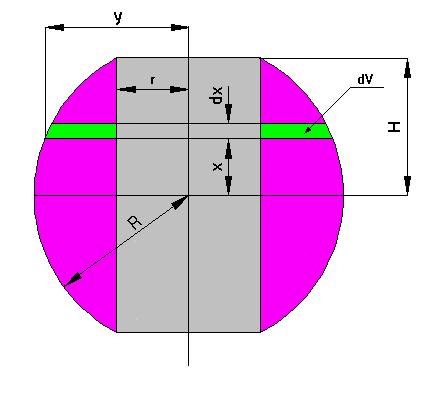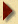If we call H the dimension from the centre of the sphere to one of the flat ends, R the sphere radius, r the cylindrical hole radius and x the distance from the centre of the sphere to some plane normal to the cylinder axis (refer attached picture). This plane intersects the sphere at a distance y from the axis.

Using Pythagoras's theorem we have:

r2 = R2 - H2
y2 = R2 - x2

The volume dV between the plane at x and that at x + dx is :

dV = PI * ( y2 - r2 ) * dx

Replacing y2 and r2 by their value above, we get:

dV = PI * ( H2 - x2 ) * dx

Obviously this shows that the volume is independent of R and depends on H only, if one makes R decrease to H then the internal cylinder decreases in volume and at the limit it becomes 0 when the sphere radius is equal to H

The answer therefore is V = 4/3 PI * H3 . It is also the simplest way I know to prove that the volume of a sphere is 4/3 PI * R3, simply integrate dV (very simple) then make H tend to R.

V = 36 Pi cubic centimetres.Back to Puzzle Page